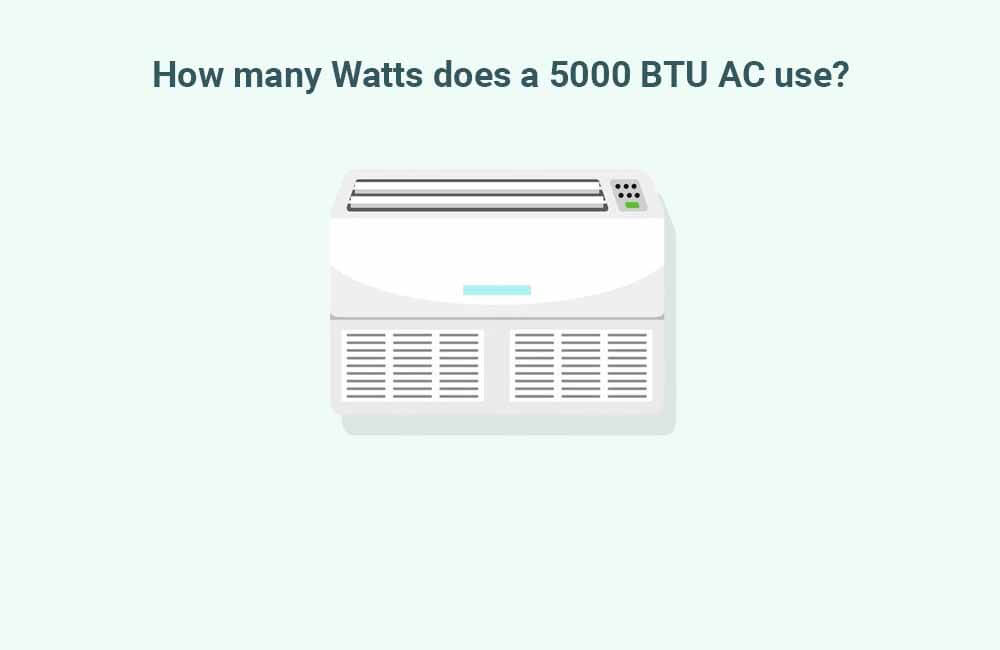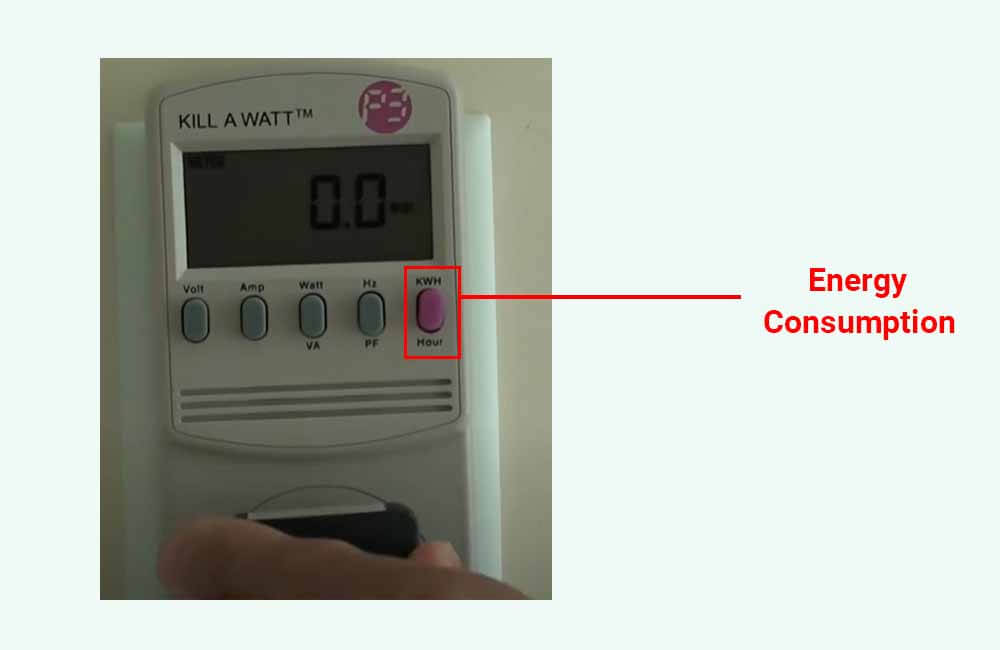# How many watts does a 5000 BTU AC use?

When it comes to a 5000 BTU air conditioner, the BTU rating signifies its heat exchange capacity. However, the actual power required to achieve this level of cooling depends on the unit’s efficiency, which is quantified by its Energy Efficiency Ratio (EER).

Consequently, the wattage usage of different 5000 BTU air conditioners varies depending on their specific models.

In this article, I will guide you through the process of determining the power usage of your 5000 BTU window air conditioner, while also addressing other important aspects of its electrical consumption, such as its amp usage and kWh usage

Let’s dive in.## How many watts does a 5000 BTU AC use?

On average, a 5000 BTU air conditioner typically uses around 450 to 500 Watts of power when it’s running. At 115 Volts, these units draw approximately 4 amps of current (which is about 40 amps at 12 Volts). However, some high-efficiency models with an EER (or CEER) rating of 12 or higher can consume as little as 350 Watts of power.

To determine the specific wattage of your 5000 BTU AC unit, you have a few options. The simplest approach is to locate the technical specification label, usually attached somewhere on the unit itself.

On the specification label, keep an eye out for the Power (wattage) rating specified in Watts (W). It might be listed as Watts, Rated Input, Power Input, Rated Power, or a similar term.

For example, here’s a specification sticker of a 5000 BTU unit that explicitly specifies 455 Watts as the power usage of the unit:In some cases, the specifications label does not include the exact power usage of the unit in Watts, but instead provides the Amperage and Voltage of the air conditioner:

• The Amperage: This rating is measured in Amps (A) and is usually labeled as Amps, Current, Rated Amps, Rated Current, Current Input, etc…
• The Voltage: This rating is measured in Volts (V). In the U.S., appliances are generally rated at 115-130 Volts.

At 115 Volts, the Amperage of a 5000 BTU unit should be between 3.5 and 5.5 Amps. Usually, these units are rated at around 4 Amps. Once you find the Amps and Volts of your unit, you can calculate the power rating of your unit via the following formula:

Watts = Amps x Volts

For example, if you look at the specifications label above, you can see that the manufacturer specifies 4 Amps as the rated current of the unit and 115 Volts as its Voltage. The Wattage of the unit can then be calculated as such:

Power Usage (Watts) = Amperage (Amps) x Voltage (Volts)

Power Usage (Watts) = 4 Amps x 115 Volts

Power Usage (Watts) = 460 Watts

Now, there is a 5 Watt difference between the Rated Power Usage of the unit (455 Watts) and the Power Usage we’ve calculated using the AC’s Amperage and Voltage; however, this small variation is quite common. It can be attributed to factors such as measurement tolerances, rounding of values, or slight variations in the actual operating conditions of the appliance.

Another common way to estimate the power usage of your 5000 BTU air conditioner is to use the EnergyGuide (yellow) label that comes with the AC unit. In the label, you’ll find an Energy Efficiency Ratio (EER), or a Combined Energy Efficiency Ratio (CEER) for new units.

You can then calculate the rated wattage of your 5k unit by dividing its capacity by the provided CEER (or EER) rating:

Power Rating (Watts) = Capacity (BTU)  ÷ CEER (or EER)

For example, here’s an EnergyGuide label that specifies a Combined Energy Efficiency Ratio (CEER) of 11:The Power Usage of this particular unit can be calculated as such:

Power Rating (Watts) = Capacity (BTU)  ÷ CEER (or EER)

Power Rating (Watts) = 5000 BTUs  ÷ 11

Power Rating (Watts) = 454.5 Watts

If none of these labels is provided, and you can’t find the electrical specification of your unit on the web, you can use an electricity monitoring device such as the Kill-A-Watt or the Poniie meters.

You can use one of these devices by plugging it into the wall socket (or the inverter) and plugging your air conditioner into the device. The electricity meter can then display the exact power usage of your unit.

Take a look at this video showcasing a 5000 BTU window unit connected to a Kill-A-Watt meter:

In the video, you can see that the wattage readings from the Kill-A-Watt show a Power Usage of about 480 Watts.

However, you’ll also notice that when the unit is restarted, it briefly experiences a power surge, drawing slightly more than 2000 Watts. This brief surge in power usage is a common occurrence, as most air conditioners often require a higher initial power draw during startup.

This startup wattage reading is particularly useful when trying to size an inverter that can run your air conditioner.

Now that we’ve discussed the power usage of your 5000 BTU AC unit, let’s talk about its energy consumption (kWh), which is an even more important aspect of the electricity usage of your unit.

## How much energy (kWh) does a 5000 BTU air conditioner use?

While Power is measured in Watts or kiloWatts, and represents the rate at which electricity is consumed, Electrical Energy is measured in Watt-hours (Wh) or kiloWatt-hours (kWh) and represents the actual quantity of electricity that’s been consumed over a certain period of time.

On average, a 5000 BTU air conditioner will generally use between 300 and 400 Watt-hours of energy per hour, which equates to between 0.3 and 0.4 kWh per hour. The exact energy usage of these window units will depend on a few factors, such as ambient temperature, the efficiency of the unit, and the quality of the insulation.

The following table provides estimates of the energy consumption of 5000 BTU air conditioners based on their run time:

You can actually estimate the energy consumption of your 5000 BTU window unit using its Wattage (Watts), and Usage Duration (hours). Let me explain.

The energy consumption of most appliances can be calculated as such:

Energy Consumption (Wh) = Power Usage (W) x Usage Time (hours)

And:

Energy Consumption (kWh) = Energy Consumption (Wh) ÷ 1000

A simple example:

Let’s consider a light bulb that is rated at 50 Watts. If this light bulb is left on for 5 hours, the amount of energy that it consumes over those 5 hours can be calculated as such:

Energy Consumption (Wh) = Power Usage (W) x Usage Time (hours)

Energy Consumption (Wh) = 50 Watts x 5 hours

Energy Consumption (Wh) = 250 Watt-hours

Energy Consumption (kWh) = Energy Consumption (Wh) ÷ 1000

Energy Consumption (kWh) = 250 Wh ÷ 1000

Energy Consumption (kWh) = 0.25 kWh

A light bulb rated at 50 Watts, when left on for 5 hours, will consume 250 Watt-hours (0.25 kWh) of energy.

However, it’s important to note that calculating the exact energy consumption of a window AC unit is more complex.

Unlike a light bulb that remains consistently powered, an air conditioner’s compressor may not run continuously when the unit is left on. This variability makes it more difficult to determine the precise energy consumption of an air conditioner without additional information or monitoring capabilities.

Most 5000 BTU air conditioners are of the non-inverter type, which means they run at full capacity when they’re turned on, and the compressor automatically turns off after the set temperature is reached. After that, these units will turn ON and OFF in a way that maintains that set temperature.

The percentage of time for which the air conditioner is really ON is referred to as the Duty Cycle (%). This duty cycle will mainly depend on how easy it is to achieve and maintain the temperature setpoint.

As a result, the energy consumption formula becomes:

Energy Consumption (Wh) = Power Usage (W) x Usage Time (hours) x Duty cycle (%)

For example, when initially cooling down a room, a 5000 BTU AC unit may run nearly 100% of the time and consume about 400 to 450 Watt-hours of energy in the first hour. Once the room reaches the desired temperature, the unit may only run for 50-80% of the time, consuming approximately 200-350 Watt-hours per hour.

As a general guideline, over a 5-hour period, you can assume an average duty cycle of around 70%. However, the duty cycle can vary depending on factors like room temperature and insulation quality. For instance, higher temperatures may result in a higher duty cycle, while better insulation can reduce the duty cycle.

As a rough estimate, temperatures hovering around 90°F tend to correspond to a duty cycle of approximately 75%, whereas temperatures surpassing 95°F with average insulation may result in a duty cycle of around 90%.

For example, let’s say that the AC is rated at 450 Watts, and let’s use 80% as our duty cycle. We can estimate the energy consumption of our AC over 5 hours as such:

Energy Consumption (Wh) = Power Usage (W) x Usage Time (hours) x Duty cycle (%)

Energy Consumption (Wh) = 450 Watts x 5 hours x 80 %

Energy Consumption (Wh) = 1800 Watt-hours

Energy Consumption (kWh) = Energy Consumption (Wh) ÷ 1000

Energy Consumption (kWh) = 1800 Wh ÷ 1000

Energy Consumption (kWh) = 1.8 kWh

According to our calculations, this 450W AC unit would consume 1800 Watt-hours (1.8 kWh) of energy over 5 hours, which is equivalent to about 360 Wh/hour (0.36 kWh/hour).

However, keep in mind that each situation is unique, and Factors like room layout, insulation effectiveness, and personal comfort preferences can all impact the actual duty cycle experienced in practice.

To obtain more accurate and precise measurements of your 5000 BTU air conditioner’s energy consumption, using an electricity monitoring device (such as the Kill-A-Watt meter) is recommended.

To measure the energy consumption of your air conditioner over a specific period, such as 8 hours of daily usage, simply plug the air conditioner into the wall outlet (or inverter) via the Kill-A-Watt meter. The meter will provide you with the exact amount of energy consumed by the air conditioner during that time period.After turning your AC on, push the button that says KWH (purple button), and the device will display the energy consumption.

### How much battery power is needed to run a 5000 BTU air conditioner?

The amount of battery power required to run these units will depend on their usage time and the energy they consume during this time. But it will also depend on the type of batteries you’ll be using.

For example, to run a 5000 BTU AC unit for 3 to 4 hours, you would need a 12V-100AH lithium battery or 2 12V-100AH lead-acid batteries. To run it for 7 to 8 hours, you would need to double the amount of battery capacity.

### Will a 3000 watt inverter run a 5000 BTU AC?

A 3000 Watt inverter can run a 5000 BTU air conditioner. However, unless the inverter is running other appliances as well, its efficiency is likely to be very low. If the 5000 BTU unit is the only load running on the inverter, the inverter should be rated at 1500-2000 Watts.

It is also important to note that the inverter should be of the Pure Sine Wave type.

### How many solar panels to run 5000 BTU AC?

The exact amount of solar power that you need to run a 5000 BTU air conditioner will depend on the daily energy consumption of the unit, and on your location.

Assuming it runs for 8 hours a day, as a general rule of thumb, you would need 600 Watts of solar power to run a 5000 BTU air conditioner. However, you would also need a solar charge controller, a battery bank, and an inverter.##### Younes

Hi! My name is Younes. I'm an electrical engineer and a renewable energy enthusiast. I created renewablewise.com with a mission of delivering digestible content and information to the people who seek it.